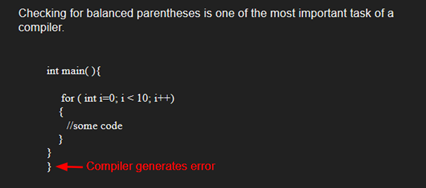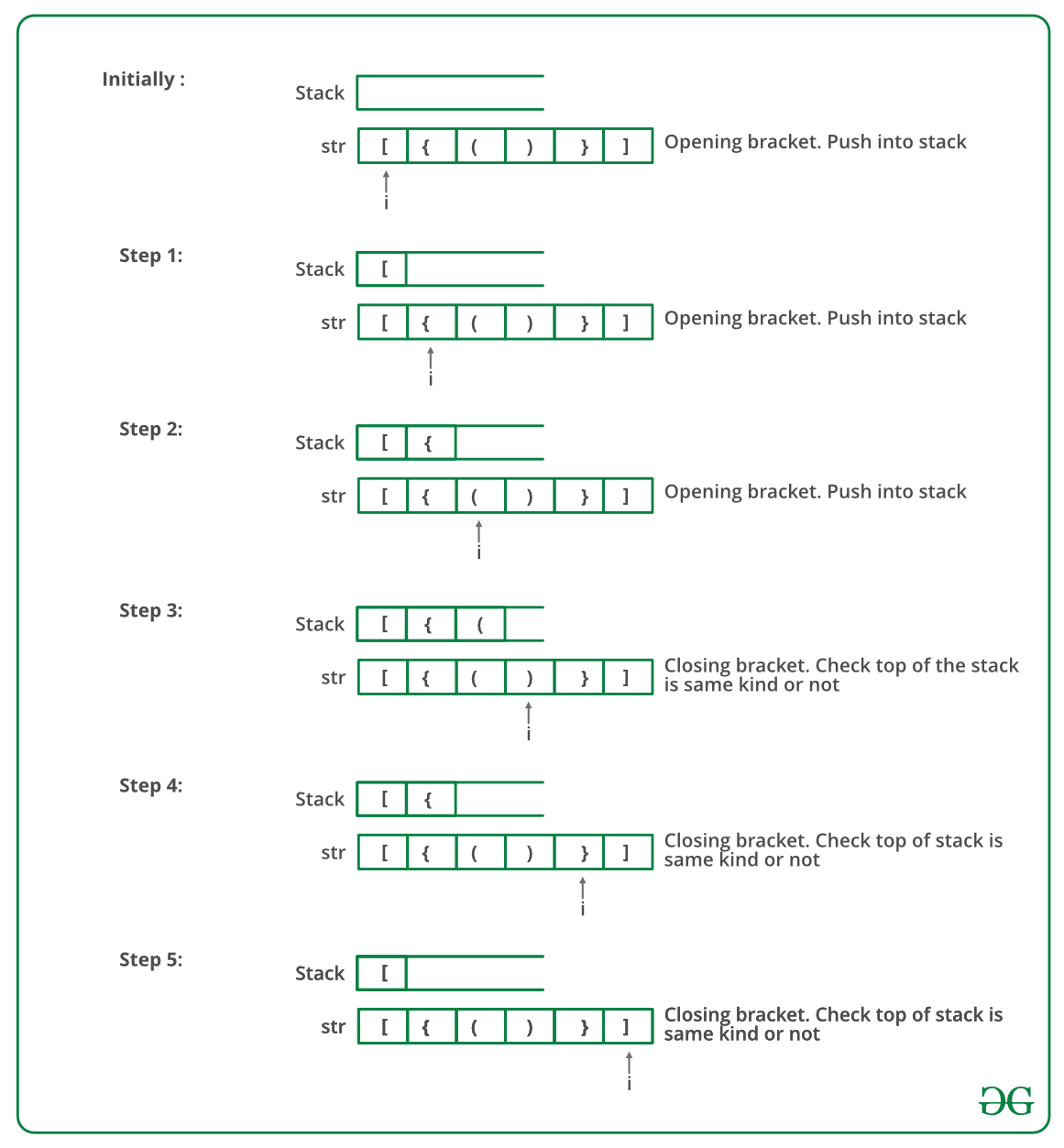GeeksforGeeks App
Open AppBrowser
Continue

# C++ Program To Check For Balanced Brackets In An Expression (Well-Formedness) Using Stack

Given an expression string exp, write a program to examine whether the pairs and the orders of “{“, “}”, “(“, “)”, “[“, “]” are correct in exp.

Example

Input: exp = “[()]{}{[()()]()}”
Output: Balanced

Input: exp = “[(])”
Output: Not BalancedAlgorithm:

• Declare a character stack S.
• Now traverse the expression string exp.
1. If the current character is a starting bracket (‘(‘ or ‘{‘ or ‘[‘) then push it to stack.
2. If the current character is a closing bracket (‘)’ or ‘}’ or ‘]’) then pop from stack and if the popped character is the matching starting bracket then fine else brackets are not balanced.
• After complete traversal, if there is some starting bracket left in stack then “not balanced”

Below image is a dry run of the above approach:Below is the implementation of the above approach:

## C++

 `// CPP program to check for balanced brackets.``#include ``using` `namespace` `std;`` ` `// function to check if brackets are balanced``bool` `areBracketsBalanced(string expr)``{  ``    ``stack<``char``> s;``    ``char` `x;`` ` `    ``// Traversing the Expression``    ``for` `(``int` `i = 0; i < expr.length(); i++) ``    ``{``        ``if` `(expr[i] == ``'('` `|| expr[i] == ``'['``            ``|| expr[i] == ``'{'``) ``        ``{``            ``// Push the element in the stack``            ``s.push(expr[i]);``            ``continue``;``        ``}`` ` `        ``// IF current current character is not opening``        ``// bracket, then it must be closing. So stack``        ``// cannot be empty at this point.``        ``if` `(s.empty())``            ``return` `false``;`` ` `        ``switch` `(expr[i]) {``        ``case` `')'``:``             ` `            ``// Store the top element in a``            ``x = s.top();``            ``s.pop();``            ``if` `(x == ``'{'` `|| x == ``'['``)``                ``return` `false``;``            ``break``;`` ` `        ``case` `'}'``:`` ` `            ``// Store the top element in b``            ``x = s.top();``            ``s.pop();``            ``if` `(x == ``'('` `|| x == ``'['``)``                ``return` `false``;``            ``break``;`` ` `        ``case` `']'``:`` ` `            ``// Store the top element in c``            ``x = s.top();``            ``s.pop();``            ``if` `(x == ``'('` `|| x == ``'{'``)``                ``return` `false``;``            ``break``;``        ``}``    ``}`` ` `    ``// Check Empty Stack``    ``return` `(s.empty());``}`` ` `// Driver code``int` `main()``{``    ``string expr = ``"{()}[]"``;`` ` `    ``// Function call``    ``if` `(areBracketsBalanced(expr))``        ``cout << ``"Balanced"``;``    ``else``        ``cout << ``"Not Balanced"``;``    ``return` `0;``}`

Output

`Balanced`

Time Complexity: O(n)
Auxiliary Space: O(n) for stack.

Please refer complete article on Check for Balanced Brackets in an expression (well-formedness) using Stack for more details!

My Personal Notes arrow_drop_up